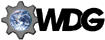# Guide to frames usage

## Frames syntax: the FRAMESET element

A frameset document uses the FRAMESET element instead of the standard HTML element BODY. The element specifies either a series of rows or columns. Inside the FRAMESET opening and closing tags, each row or column is set up using the FRAME element. To set up a combination of columns and rows, use FRAMESET instead of FRAME.

### Syntax for FRAMESET

The FRAMESET element has required opening and closing tags. It has two attributes, ROWS and COLS. One, and only one, of these must be used in every FRAMESET tag. The syntax for both attributes is the same, but using ROWS defines a series of rows, and COLS defines a series of columns.

### Defining rows or columns

In the ROWS or COLS attribute, the width (or height) of each row or column is specified as a series of comma-separated values. A value can be given in pixels or as a percentage. Percentages are given as n%, where n is a number between 0 and 100. Numbers are assumed to be pixel values. Relative values can also be given, using the special `*` value. The width or height of columns whose value is given using `*` is determined after pixel width and percentage widths have been allocated.

Using `*` just once means "remaining space should be assigned to this frame." If it is used multiple times in a series, the remaining space is divided equally between all the frames in question. If a number is put before the `*`, then it is interpreted as a relative weight.

#### Examples

`<FRAMESET ROWS="100,200">`
This specifies two rows. The first row (upper row) is 100 pixels high and the second, lower row is 200 pixels high. If the display is more than 300 pixels high, the rows are both resized proportionally.
`<FRAMESET COLS="50%,50%">`
Here two equal-sized columns are specified. This is equal to `<FRAMESET COLS="*,*">`.
`<FRAMESET COLS="33%,33%,*">`
This is the recommended way to specify three columns. If `COLS="33%,33%,33%"` was used instead, there would have been a 1% of screen space unallocated, which would mean that one (randomly chosen) column would be slightly wider. Because now `*` is used for the third column, this one will be slightly wider.
`<FRAMESET ROWS="100,*,2*">`
Three rows are defined. The first one is 100 pixels high. The height of the other two is determined next. The remaining space is divided into three portions, of which the second row gets one, and the third row gets two.
Note that, if the values given do not match the browser window sizes, or are invalid (for example, a series of percentages that exceeds 100) the browser resizes all values proportionally to make them "fit".Last modified: 27 Apr 1997.
Feedback: galactus@htmlhelp.com
Copyright © 1996 - 2006. Arnoud "Galactus" Engelfriet. All rights reserved.